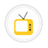you are here->home->Mathematics->Class 9
Class 9
These activities are part of the class IXth syllabus under CBSE curriculamProperties of parallelogramTo explore similarities and differences in the properties with respect to the diagonal of a parallelogram.Area of circleTo show that the area of circle is half the product of its circumference and radius.Solving simultaneous equationsTo solve simultaneous linear equations in two variables using LCM method and substitution method.Circumcentre of a triangleTo show that the perpendicular bisectors of the sides of a triangle concur at a point (called the circumcentre)Area of triangleTo show that the area of a triangle is half the product of the base and the height.The quadrilateral formed by the mid-points of a quadrilateralTo show that the figure obtained by joining the mid points of consecutive sides of the quadrilateral is a parallelogram.Area of trapeziumTo show that the area of trapezium is equal to half the product of its altitude and sum of its parallel sides.UI TemplateMaths templateIncentre of a triangleTo show that the internal bisectors of the angles of a triangle concur at a point (called the incentre), which always lies inside the triangle.Mid-point theoremTo show that the line segment connecting the mid-points of two sides of a triangle is parallel to the third side and is congruent to one half of the third side.Centroid of a triangleTo show that medians of a circle concur at a point (called centroid) and which always lies inside the triangle.Area of rhombusTo show that the area of rhombus is half of product of its diagonals.Area of parallelogramTo show that the area of parallelogram is product of its base and height.